Estimate Reconciliation and Probability

Summary
A/E, CMs and independent estimators are being asked to deliver deterministic estimates based on data that is inherently probabilistic. The difference between the 50% confidence level and the 90% confidence level may be very large.  A/Es and Owners may be happy to estimate to the 50% certainty, while the CM and Subs could not stay in business if they only made a profit on 50% of their projects.  Estimates given as definite numbers (“point estimates”) are misleading, since all estimates of future events are uncertain.  Estimates given as a probability lead to a statement regarding the confidence in a number, rather than a definite number.  Some managers find probability statements less useful, despite their inherent logic.  However failure to recognize the probabilistic nature of estimates may lead to management errors.  Reconciliation of estimates is misleading, if one party has high confidence in an estimate or low risk if the estimate is incorrect, while the other party has low confidence in the estimate or high risk if the estimate is incorrect.  A cost estimate at the 50% confidence level, which is a satisfactory level of confidence by an Owner or A/E, may be quite different than one at the 90% confidence level, which is satisfactory to a contractor. This is illustrated in an example that demonstrates a cost difference of 15% between two levels of confidence.

Report
The CMAR survey revealed differences in the opinions of the parties regarding estimates - especially regarding the value of a third party estimator and the value of reconciling estimates during preconstruction.  In the original report I note the different point of view of the parties about the estimate and the risk involved with the estimate and how this might affect their attitude and their numbers.  Here I hope to improve communications about estimating by combining a little theory about estimating risk with some practical insights I gleaned in 20-plus years of construction, A/E, and project management experience.  (Your comments are welcome, since all that expertise has taught me I can be mistaken.)

Estimates are a statement about a future event; such statements are also known as “divining.”   The diviners have seen a lie, and have told false dreams; they comfort in vain (Zechariah10. 2).  (Old Zechariah must have known my financial analyst.) Or as Master Yoda explained, “The future, cloudy it is.”  The Romans had an official, known as the augur, whose job it was to divine the future of Rome’s ventures, especially military.  He would take a pigeon, flop it on its back, cut out the entrails and from their shape determine if the venture should go forward.  Old Augie was on the spot and had to decide whether to war or not, unlike the Oracle at Delphi who could give answers that might be interpreted several ways.

So, realize that “estimates” are essentially guesses and often have a serious downside if they are wrong.  The reason they are guesses is that there are future events (“states of nature”) that are uncontrollable and these events will control the outcome.  Regarding what we can say about these future events, there is a continuum.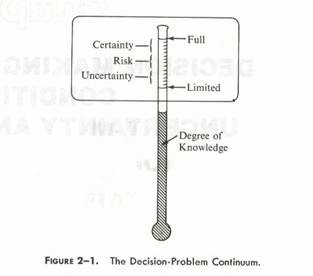Fig. 2-1 pg 48 Introduction to Management Science, Thornton, B.M. and Preston, P., Charles E. Merrill Publishing Company 1977

If we have full knowledge (we believe) of the future, we refer to that a “certainty.”  We might approximate that if we have a firm quote from a bonded sub or supplier.  Many estimating decisions are made under “risk.”  In technical terms, “risk” means we feel we can state the probability of the events.  For example, we know the price of concrete in the summer is likely to be \$200/CY but may vary by 15%.  At the other end of the knowledge spectrum we have “uncertainty.”  We recognize alternate states of nature may happen, but we don’t have a clue how likely they are.  Note the difference between the technical use of those terms and the common usage.   While the entire future is “uncertain,” if we feel confident we know the probability of the future we say there is “risk” and limit the use of “uncertain” to situations where we do not know the probability of events.  Virtually all estimating decisions involve risk.  We account for uncertainty with contingency, that is, things will happen (usually bad), but we don’t know what they will be or what they will cost.

Say we are building a road and plan to complete before freeze up.  If we don’t complete we will need to demobilize this winter and remobilize next spring – a costly matter.  We can get climate records and determine the historical dates of freeze up and we can call our friend at the weather service and get her estimate of conditions this year.  From that we can state the probabilities of freeze up by a particular date, say “70% of time we can work until October first.”  Thus we examine the risk of planning to work until October 1.  On the other hand, there is a chance our key foreman will quit, although he has been with us 20 years and we have no indication he is unhappy.  Such events are uncertain and we generally cannot estimate them.  So the freeze up date is a risk, while the foreman quitting is one example of a jillion uncertainties.  One of my favorite old superintendentism is, following a string of minor unexpected disasters, “Cheer up.  I’ve seen things go on like this for weeks and suddenly take a turn for the worse.”

Now we’ll consider some details of a “final estimate” or bid.  All of what follows could be said of planning estimates, rough order of magnitude estimates, etc. Although we can’t tell the future, the bids are due by Thursday, and I must have a number for the installed cost of a wrought iron circular stair the architect dreamed up.  I’ve never seen such a thing, much less estimated one.  Since that is only a small component of the project, my boss wants me to give her “a number” that she will input into a spread sheet with dozens of other numbers.  Since I am giving one number, it is called a “deterministic” estimate.

Deterministic
A deterministic estimate is one number for the item being estimated. It’s also called a “point estimate,” “most likely estimate,” “precise estimate,” and perhaps other names as well.  My boss does not want a discussion of pigeon guts - just a number.  I might arrive at the number several ways.  If I have experience in the item, I may just have a number I am comfortable with.  It is simply my “personal opinion” or “expert opinion.”  Often, when expressing a personal opinion estimate, I will add something to my true estimate, sometimes called a “factor of safety” or “lanyap” or some such cute euphemism.  This addition may be conscious or subconscious.  We have all discovered that the penalties for underestimating are much greater than the rewards for overestimating.  Of course I might ask the guy in the next cube’s advice, but again, that is an opinion.  We recognize that we have converted uncertainty or risk from our viewpoint into certainty for our boss’ estimate.  Note she is not certain of the outcome either, but she is certain that “This is the number Perkins gave me.”

Sometimes estimates are made by a committee or “jury of expert opinion.”  There is lots of management research about such committee’s deliberations.  Often a boss will attend the meeting and that brings up the domineering effect of one person on the committee.  There are several methods, some known as “Delphi methods,” of eliminating this effect.  But when the committee is done, they will either give a number to a deterministic estimate, discussed above, or parameters to other estimates, our next topic.

Non-deterministic to deterministic
Non-deterministic estimates recognize that there are many possible outcomes.  First we discuss methods that convert these to one number.

Expected Value
If I know the probabilities of future events and the events are mutually exclusive I can state them as such.  Suppose several suppliers have issued qualified quotations, with a “while supplies last” caveat.  I discuss this with the suppliers and determine the probability I will be able to use the suppler and the cost is in the table

 Probability Cost P * C Supplier A 50% \$35 \$17.50 Supplier B 30% \$40 \$12.00 Supplier C 20% \$65 \$13.00 Total 100% Expected Value \$42.50

Note the probabilities must add up to one.  Now I can go ahead and use the Expected Value, \$42.50, as if it were a point estimate.

Another technique for converting probabilistic data (guesses) into a number is the beta distribution.  One uses an opinion, guess, or committee to provide three numbers by asking, “What is highest/lowest/most likely cost of the item.  A good definition of “highest” is, the number that you are almost/95% sure the actual number will be less than, and similarly for the lowest.  Those three numbers are then plugged into the formula

Point estimate = (Highest estimate + 4*Most likely + Lowest estimate)/6

Although this looks flakey, this uses the statistical function called the beta distribution. Tests indicate that the point estimate derived this way is, on the average, better than just using the most likely number – or so the experts say.

Before we go on, we have limited ourselves to getting “one number.”  I could have reported a range of values, usually just the highest and lowest, if that is what my boss wanted, but the principles would be the same.

Non-deterministic
Non-deterministic estimating does not return a number, but gives a probably of certain numbers, such as, “I am 95% sure the cost will be less than \$50.00,” or “We are 60% sure the cost will be between \$30 and \$45.”  While such expressions of probable cost are tedious and seem fuzzy compared with “I estimate the cost will be \$45,” in fact it is the probability that is accurate while the precise estimate is a guess.

Say my estimate of the wrought iron stair costs has these components:

 Item Unit Cost Units (hr) Extended Buy stairs \$5000 \$5000 Carpenter time \$35 40 1400 Welder time \$42 20 840 Painter time \$32 20 640 Rent crane \$120 8 960 Total \$8840

.
So we have a deterministic estimate, \$8840.  What are the chances it will cost exactly \$8840.  The answer is zero.  We will be happy if it is close to that number, but it is very unlikely it will be exactly that number.

Now let’s look at how we got the numbers.  We called the wrought iron fabricator and he told us, “it depends how busy we are, and material costs at the time you give us the PO.  It may cost anywhere between \$3500 and \$7000.”  For the trade people, I know the wage rate from the union scale and our computed burden, but how about the time – how long will it take to get the job done?  I ask the carpenter foreman when he stops by and he tells me, “it varies quite a bit, my guess is 40 hours, but it could take anywhere from 30 to 70 hours, but 40 is my best guess.”  The welder tells me it he is “pretty sure” he can complete between 15 and 25 hours.  The painter tells me the same.  The crane shop tells me they will charge me \$120 if they have a crane, but if they have to rent one for me it will cost double that.  They do say there is only a 20% chance they will have to rent, this time of year.

From that input I put together the table below.  I applied judgment to guess for each variable, what I thought it might be, and determined the highest and lowest costs for each item.  I return one number, but since it is made up of many guesses, how sure am I that the number is correct?  What I and my boss do next depends on the competitive situation.  Note the difference between the lowest and highest numbers, \$6, 600, and either high or low number could be defended.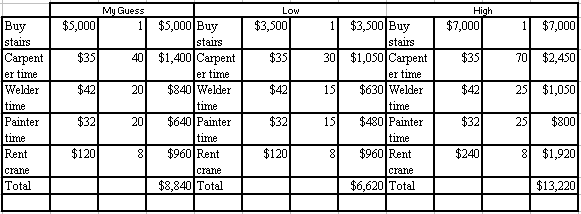Next, I can input my guess, the high, and the low estimate into a beta distribution analysis.
Point estimate = ( \$6,620 + 4*\$8,840 + \$13,220 )/6  = \$9,200
Note I could have done a similar analysis for each item, and then added them.

Simulation
A better analysis recognizes that each of the items has a factor that is unknown, but we have some idea of the probability of the various values that factor might have.  Statisticians call each of those factors a “random variable.”  For example, the amount of carpenter hours is a random variable. We put 40 hours into the estimate as if it was a number, but in fact it is not a number, but may have many values, depending on what happens in the future.  What we can put into the estimate is a “probability distribution” that states the likelihood of each value of the random variable.

Let’s consider the probability distribution of the first random variable, the cost of the staircase.  The number can be anything between the two limits and the probability is equal for all numbers within those limits.  This is called a uniform distribution.  Here is a graph of it.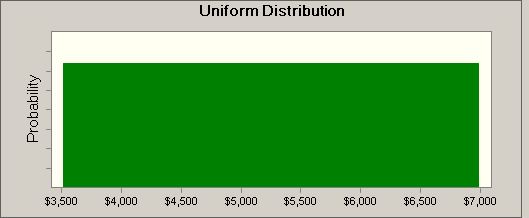The random variable of the carpenter’s time might be described by a triangular distribution.  He gave us the least, maximum, and most likely times.  Here is a graph of that: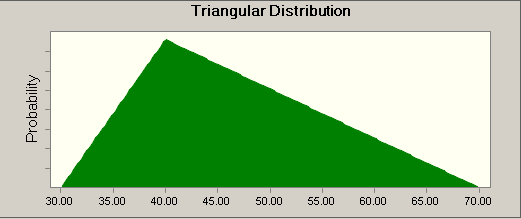You don’t see a scale on the Y axis.  But, the scale will be such that the area of the triangle is 1.0, and that is always the case with probability distributions, the area under the curve is always exactly one.

The welder and painter are similar, they have given a range that they have some confidence in, but are by no means sure.  Let’s translate the “pretty sure” into meaning that they are about 68% sure they will finish within those limits.  Of course there is some chance that it could be a lot longer, and for the moment let’s assume it could be shorter as well.  The “normal distribution” or “bell curve” has the property that 68% is the probably within one “standard deviation” of the average.   So let’s approximate the welder and painters times as a normal distribution with an average (or “mean”) of 20 hours and a “standard deviation” of 5 hours.  If I did that right and we could compute and find that about 65% of the area, that is the probability, lay between 15 and 25 hours, just as the mechanics told us.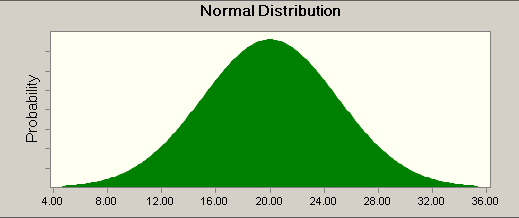Finally the crane cost has a percentage value.  This is figure is not a probably distribution, the chart just shows it will be one number 80% of the time and 20% the other.So, what you would like to do is somehow add these probabilities, somewhat like you added the probabilities above in the expected value method.  The problem is that can’t be done, except in the simplest cases.  What can be done is called “simulation” and the best known simulation method is called Monte Carlo simulation.  Unlike the trivial name, it is a very powerful technique.  Today, Monte Carlo simulations are easily done on your desktop with a program, Crystal Ball, an Excel-like program.  What Monte Carlo simulations do is throw a random number into formulas for the probability distributions of each random variable.  That process is done for all the factors in your computation.  If you only did that once, it would not make much sense, of course, but the simulation program does that thousands of times and finally returns a probability distribution of the result.

Crystal ball ran the simulation with 100,000 trails in a second or two.  Here is what it looks like: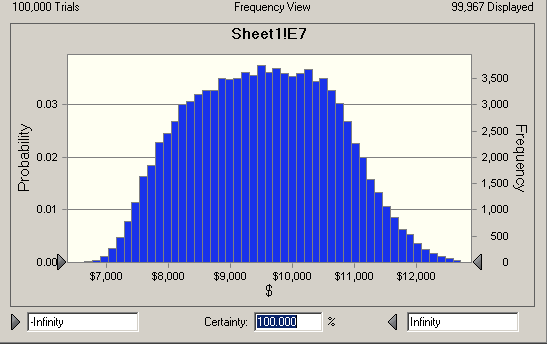Which only says the there is a 100% change the cost will be between negative infinity and plus infinity, something you could have figured out without a computer.  But now I can ask the question, what is the chance the job will cost less than my original number, \$8840?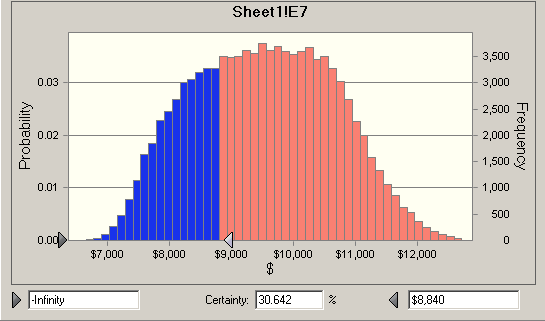So there is only a 30% chance that the job can be done for less than my original budget.

Crystal Ball lets me do show a range of outcomes, for example this shows that there is a 53% chance the job will come in between 8,000 and 10,000.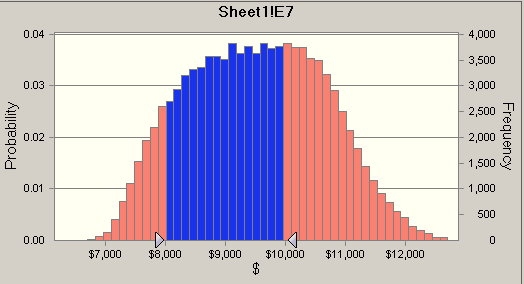It turns out the 50% level is 9548, that is, there is a 50% change the job will cost more than that number.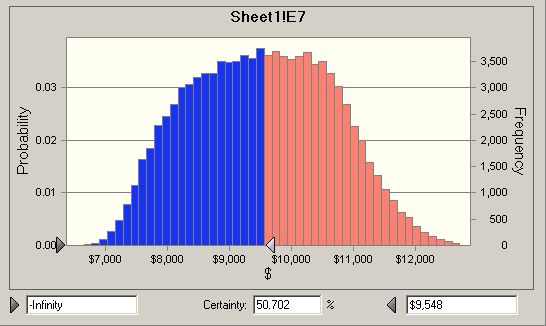Finally, there is a 90% chance the job can be done for less than \$11,000, but that means there is a 10% chance the job will cost more than \$11,000.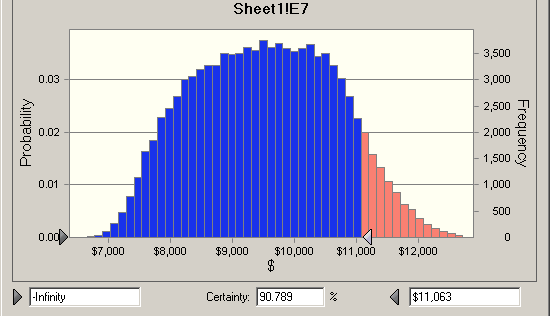Above I used the normal distribution because most of you are familiar with it.  But theoretically the normal distribution extends from positive to negative infinity.  While some tasks might seem to take forever, positive infinity, they never take less than zero time.  But the theoretical problem using the normal distribution is generally not a real problem, since values very far from the mean occur very seldom.  However there are many other probability distributions that don’t have that problem, either they start at zero or you can set the start value.  The “proper” way to do that is find the distribution that best fits your data, which we could do with freeze up date, since we have data.  Usually for estimating productivity we only have few numbers and they are never exactly the same conditions as what we are estimating.  The beta distribution and a slight variant the PERTbeta are most often used for estimating.

Estimate Reconciliation
So how can converting a simple concept, a point estimate, to a more complex concept, a probabilistic estimate, make things better?  If “better” means simpler it can't. But if better means the parties reach an understanding earlier, the answer is “probably.”  Let’s look at all the numbers in one table:

 Method Number %  Difference from point estimate Point Estimate \$8,840 - Range Low Estimate \$6,620 - 25% Range High Estimate \$13,220 49% Beta \$9,200 4% 50% Confidence \$9,548 8% 90% Confidence (less than) \$11,000 24%

We are tempted to look at the point estimate and consider it the “right number,” then judge that the beta and 50% confidence level are closest to being correct.  But of course the point estimate itself is unlikely to be exactly correct.  My point here is that the difference between the 50% confidence number and the 90% confidence number is \$1500; the 90% confidence number is 15% greater than the 50% confidence number.  Although I made the numbers up, they are not unrealistic, and the 15% difference between the 50% confidence level and the 90% confidence level seems pretty realistic to me.  Could it be, that one of the difficulties in estimate reconciliation is the level of confidence that the parties are requiring – even if none of the parties have examined the matter non-deterministically, they subconsciously perform such an analysis?

Of course real-life estimating has many complications not discussed above such as level of estimate: basic or summary; project stage: planning, rough order of magnitude; type of estimate: labor and materials, cost factor.  However the non-deterministic analysis would be appropriate for any of these.  One important concept is that of “variables.”  In many estimating situations, some costs are “fixed,” for example a quote from a reputable sub or supplier is generally fixed, while self-performed work is variable.  Hence in early CMAR estimating, all the costs are variable, while later, after award of key subcontracts, many of the costs are fixed.  Also, in a non-Murphy’s Law world, a chief estimator could take all the component estimates and assume that the overestimates and underestimates would balance out.  But remember that the estimate only dealt with probabilities we could state, future events that we know might occur and we could describe the probability of their occurrence.  But we live in a world where Murphy ’s Law is always operative, and events will happen that we did not expect or allow for.  Hopefully they will be minor, but they will occur, and that brings up the idea of contingency.

Up to this point we have only been talking about costs, not price, which would include overhead and profit.  How about the risk? Should the allowance for “risk” be placed in the “profit” category, or should one place it in a separate category for “contingency?” (And what is our departure point for pricing risk – 50% confidence or 90% confidence?) Here all but the most naïve recognize that if one has a line item for contingency, if some of the costs are driven up by the actions of third parties, they have the argument, “We didn’t damage you because you had an allowance for that in your contingency line.”  You also realize that if you put the contingency in the line item costs, they tend to get "lost" for cost management purposes.  And finally, if you put it in profit, you are unlikely to get the job.  So there is no way to get there from here.  Actually, my point is that you (the estimator) need to know where the contingency is.  How you handle it in practice might depend on the contracting and competitive situation.  In a competitive bid, there is no reason not to put the entire contingency into your profit line.  In a negotiated situation, it is OK to put it as a line, which invites frank discussion with the owner’s representative about the major uncertainties, some of them might be removed by contract provisions.  Lastly for CMAR work, some allowance must be made for risk in early stages of the estimate and the transfer of this risk into contingency as the subcontractors and other prices become firm.

So, even if you don’t run out and buy Crystal Ball and start non-deterministic estimating tomorrow, the concept is important. (Crystal Ball is quite easy to learn and has many uses – a “better mousetrap” as far as I am concerned.)  A/E, CMs and independent estimators are being asked to deliver deterministic estimates based on data that is inherently probabilistic. The difference between the 50% confidence level and the 90% confidence level may be very large.  A/Es and Owners may be happy to estimate to the 50% certainty, while the CM and Subs could not stay in business if they only made a profit on 50% of their projects.